## Example Questions

### Example Question #144 : Integers

Quantitative Comparison:

Column A

|–3 + 4|

Column B

|–3| + |4|

Column A and B are equal

Column A is greater

Cannot be determined

Column B is greater

Column B is greater

Explanation:

The operations in the absolute value are always done first. So in Column A, |–3 + 4| = |1| = 1.  In Column B, |–3| + |4| = 3 + 4 = 7.

### Example Question #1 : How To Find Absolute Value

Quantitative Comparison

|x – 3| = 3

Quantity A: x

Quantity B: 2

The relationship cannot be determined from the information given.

The two quantities are equal.

Quantity B is greater.

Quantity A is greater.

The relationship cannot be determined from the information given.

Explanation:

It's important to remember that absolute value functions yield two equations, not just one. Here we have x – 3 = 3 AND x – 3 = –3.

Therefore x = 6 or x = 0, so the answer cannot be determined.

If we had just used the quation x – 3 = 3 and forgotten about the second equation, we would have had x = 6 as the only solution, giving us the wrong answer.

### Example Question #146 : Integers

Quantitative Comparison

Quantity A: |10| – |16|

Quantity B: |1 – 5| – |3 – 6|

The relationship cannot be determined from the information given.

Quantity A is greater.

The two quantities are equal.

Quantity B is greater.

Quantity B is greater.

Explanation:

Quantity A: |10| = 10, |16| = 16, so |10| – |16| = 10 – 16 = –6.

Quantity B: |1 – 5| = 4, |3 – 6| = 3, so |1 – 5| - |3 – 6| = 4 – 3 = 1.

1 is bigger than –6, so Quantity B is greater.

### Example Question #147 : Integers

Quantitative Comparison

Quantity A: (|–4 + 1| + |–10|)2

Quantity B: |(–4 + 1 – 10)2|

Quantity B is greater.

The relationship cannot be determined from the information given.

The two quantities are equal.

Quantity A is greater.

The two quantities are equal.

Explanation:

Quantity A: |–4 + 1| = |–3| = 3 and |–10| = 10, so (|–4 + 1| + |–10|)2 = (3 + 10)2 = 13= 169

Quantity B: |(–4 + 1 – 10)2| = |(–13)2| = 169

The two quantities are equal.

### Example Question #1 : Absolute Value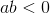Quantity A: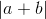Quantity B: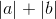Quantity A is greater

Quantity B is greater

The two quantities are equal

The relationship cannot be determined from the information given

Quantity B is greater

Explanation:

If, then eitherormust be negative, but not both. Making them both positive, as in quantity B, and then adding them, would produce a larger number than adding them first and making the result positive.

### Example Question #149 : Integers

What is the absolute value of the following equation when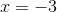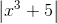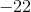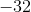Explanation:

(–3)3 = –27. Any time a negative number is cubed, it remains negative. –27 + 5 = –22. The absolute value of any number will ALWAYS be positive so the absolute value of –22 is 22. This is our answer.

Tired of practice problems?

Try live online GRE prep today.